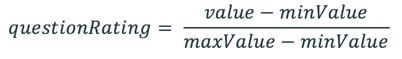Risk Assessment for Change Request- Calculation for Normalize value

Description

From the filter navigator -> Risk Assessment -> Change Risk Assessment

Assessment Categories ->  Metric Category -> Metric

Under General Tab -> Ask Question Selection

If customer creates a Metric and for Ask Question field you see " Only if script provides no default answer" the question will not show in the assessment from the UI action on the change request.

When customer uses this option , the Metric Results will show empty for the Instance column and the normalize value is calculated  with following formula

From filter navigator->asmt_assessment.list

Click on the Assessment Group -> Metric Result

Check the Instance Column to see if it is empty

Depending of the Scale definition,  if it is High or low these are the  following formulas that gets calculated

HighNormalize Value = Scale Value - minValue / maxValue-minValue

LowNormalize Value =1-( Scale Value - minValue / maxValue-minValue )

*****

If customer creates a metric  and  for Ask Question field you see " Always ask question" the question will  show in the assessment from the UI action on the change request.

From filter navigator->asmt_assessment.list

Click on the Assessment Group -> Metric Result

Check the Instance Column to see if it is empty

When customer uses this option , the Metric Results will show one with an empty instance name and one that has it.  When this happens two different calculations are running

First Calculation:

For the Metric Result that  has an instance name  it using this calculation to calculate the Normalize Value

Normalize Value =Actual_value * Weight

Second Calculation:  Script Include "ChangeRiskAsmtSNC" determining the risk for a change request

Takes the sum of the normalize values and compare the result to the threshold that was specified under the risk assessment

calcAsmtScore: function(asmtInstanceId) {

var score = 0;

var ga = new GlideAggregate(this.ASMT_METRIC_RESULT);

ga.groupBy(this.INSTANCE);

ga.query();

if (ga.next())

score = ga.getAggregate("SUM", this.NORMALIZED_VALUE);

if (this._log.atLevel(global.GSLog.DEBUG))

this._log.debug("[calcAsmtScore] score: " + score);

return score;

},

For the Metric Result  that is  showing empty for the instance name, the normalize value is calculated with the same formula as  before

Depending of the Scale definition  High or low this are the formula that gets calculated

HighNormalize Value = Scale Value - minValue / maxValue-minValue

LowNormalize Value =1-( Scale Value - minValue / maxValue-minValue )

Lastly ,  this calculation does not get factored in to the sum of normalize value because the instance name is empty.

Release or Environment

paris-06-24-2020__patch7-02-25-2021_03-03-2021_0818